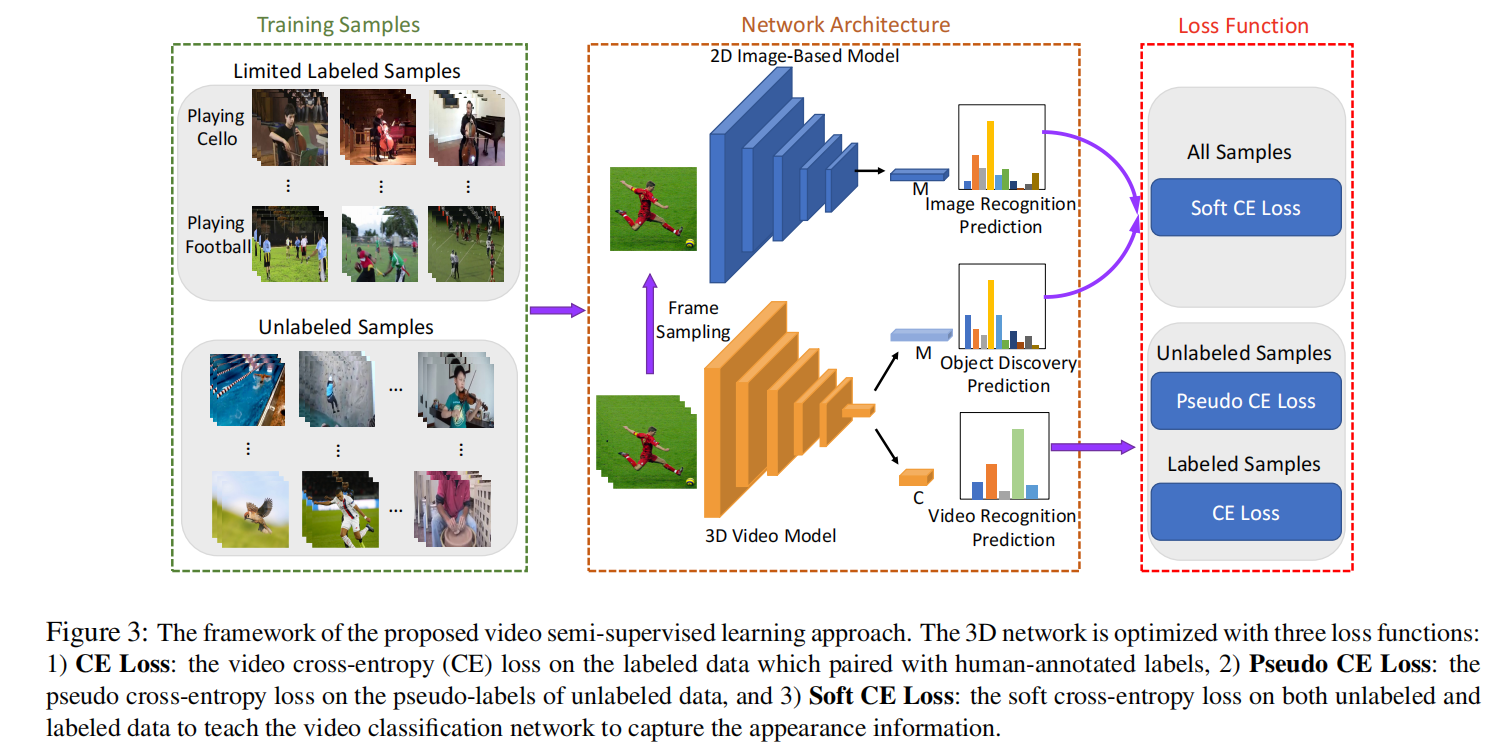# 近期看的一些论文#1

[TOC]

## Marginal Contrastive Correspondence for Guided Image Generation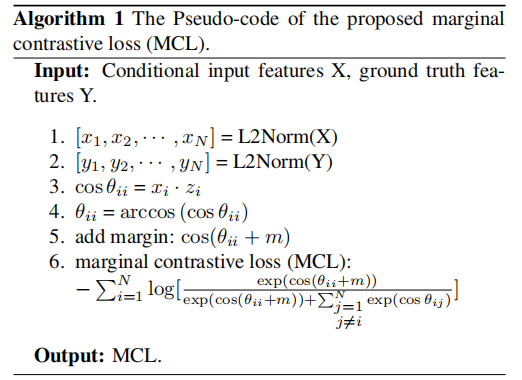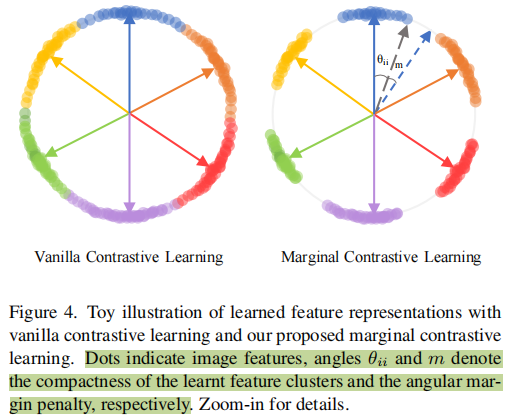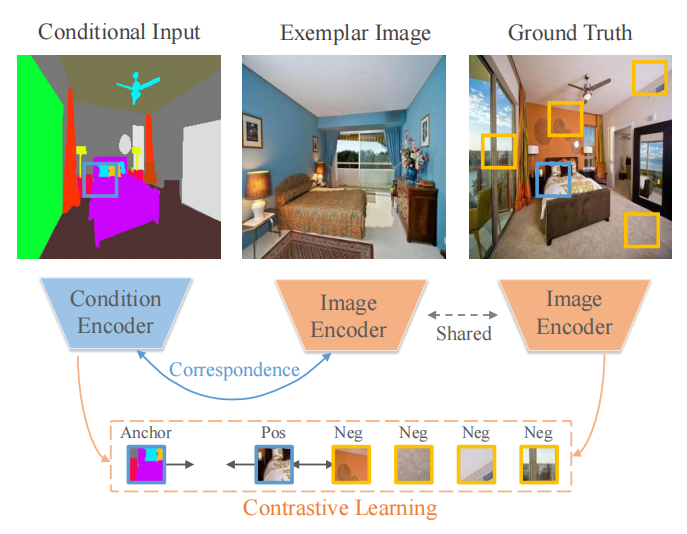## In Defense Of Pseudo-labeling: An Uncertainty-aware Pseudo-label Selection Framework For Semi-supervised Learning (ICLR 2021)

$g_c^{(i)}=\mathbb{l}[p_c^{(i)} \ge \tau_p] + \mathbb{l}[p_c^{(i)} \le \tau_n]$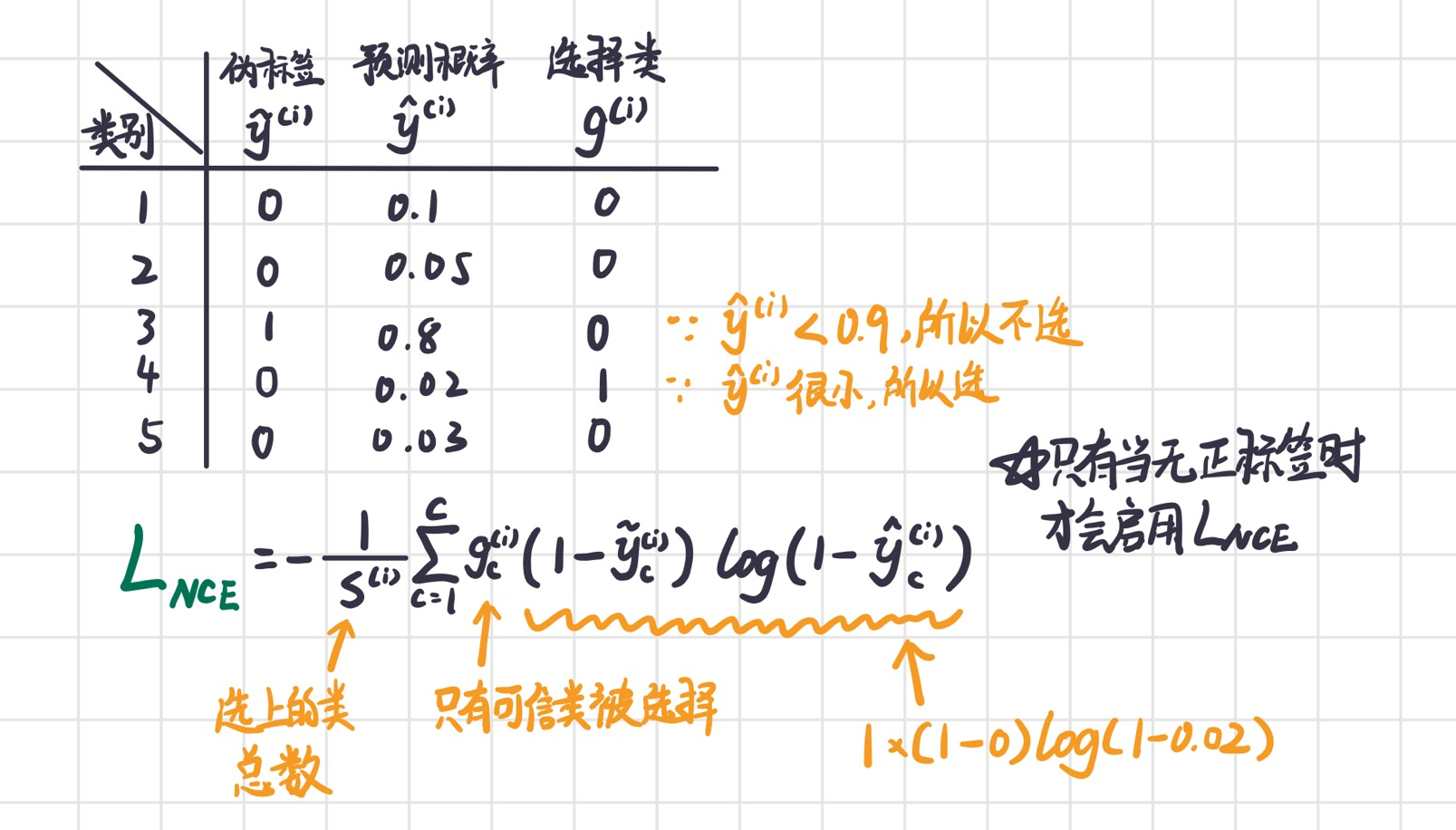$g_c^{(i)}=\mathbb{l}[u(p_c^{(i)}) \le k_p]\cdot \mathbb{l}[p_c^{(i)} \ge \tau_p] + \mathbb{l}[u(p_c^{(i)}) \le k_n] \cdot \mathbb{l}[p_c^{(i)} \le \tau_n]$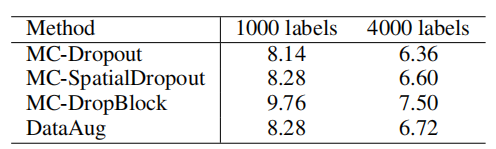## VideoSSL: Semi-Supervised Learning for Video Classification